Related Articles

# Get a list of a specified column of a Pandas DataFrame

• Difficulty Level : Basic
• Last Updated : 28 Jul, 2020

In this article, we will discuss how to get a list of specified column of a Pandas Dataframe. First, we will read a csv file into a pandas dataframe.

Example:

## Python3

 `# importing pandas module ``import` `pandas as pd ``   ` `# making data frame from csv``data ``=` `pd.read_csv(``"nba.csv"``) ``   ` `# calling head() method  ``df ``=` `data.head(``5``) ``   ` `# displaying data ``df`

Output: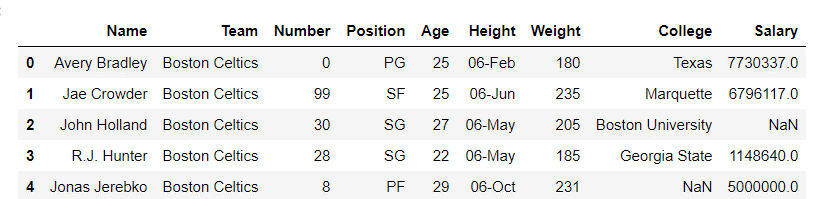Let’s see how to get a list of a specified column of a Pandas DataFrame:
We will convert the column “Name” into a list using three different ways.
1. Using Series.tolist()
From the dataframe, we select the column “Name” using a [] operator that returns a Series object. Next, we will use the function Series.to_list() provided by the Series class to convert the series object and return a list.

## Python3

 `# importing pandas module``import` `pandas as pd`` ` `# making data frame from csv``data ``=` `pd.read_csv(``"nba.csv"``)``df ``=` `data.head(``5``)`` ` `# Converting a specific Dataframe ``# column to list using Series.tolist()``Name_list ``=` `df[``"Name"``].tolist()`` ` `print``(``"Converting name to list:"``)`` ` `# displaying list``Name_list`

Output: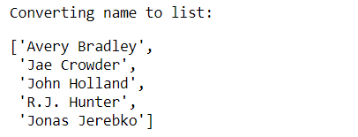Let’s break it down and look at the types

## Python3

 `# column 'Name' as series object``print``(``type``(df[``"Name"``]))`` ` `# Convert series object to a list``print``(``type``(df[``"Name"``].values.tolist()`

Output: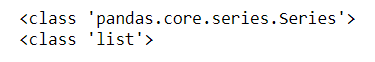2. Using numpy.ndarray.tolist()
From the dataframe we select the column “Name” using a [] operator that returns a Series object and uses Series.Values to get a NumPy array from the series object. Next, we will use the function tolist() provided by NumPy array to convert it to a list.

## Python3

 `# importing pandas module``import` `pandas as pd`` ` `# making data frame from csv``data ``=` `pd.read_csv(``"nba.csv"``)``df ``=` `data.head(``5``)`` ` `# Converting a specific Dataframe column``# to list using numpy.ndarray.tolist()``Name_list ``=` `df[``"Name"``].values.tolist()`` ` `print``(``"Converting name to list:"``)`` ` `# displaying list``Name_list`

Output:Similarly, breaking it down

## Python3

 `# Select a column from dataframe ``# as series and get a numpy array``print``(``type``(df[``"Name"``].values))`` ` `# Convert numpy array to a list``print``(``type``(df[``"Name"``].values.tolist()`

Output: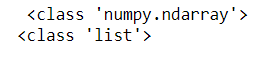3. Using Python list() function
You can also use the Python list() function with an optional iterable parameter to convert a column into a list.

## Python3

 `# importing pandas module``import` `pandas as pd`` ` `# making data frame from csv``data ``=` `pd.read_csv(``"nba.csv"``)``df ``=` `data.head(``5``)`` ` `# Converting a specific Dataframe``# column to list using list()``# function in Python``Name_List ``=` `list``(df[``"Name"``])`` ` `print``(``"Converting name to list:"``)`` ` `# displaying list``Name_List`

Output:Converting index column to list
Index column can be converted to list, by calling pandas.DataFrame.index which returns the index column as an array and then calling index_column.tolist() which converts index_column into a list.

## Python3

 `# Converting index column to list``index_list ``=` `df.index.tolist()`` ` `print``(``"Converting index to list:"``)`` ` `# display index as list``index_list`

Output: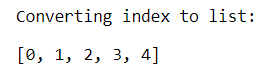Attention geek! Strengthen your foundations with the Python Programming Foundation Course and learn the basics.

To begin with, your interview preparations Enhance your Data Structures concepts with the Python DS Course. And to begin with your Machine Learning Journey, join the Machine Learning – Basic Level Course

My Personal Notes arrow_drop_up